# Doing the Math

Proper mixing and application of pesticides can be accomplished by understanding proportions, fractions, and percentages.

## Proportions and cross multiplication:

If you understand how to express your rate question as a proportion, you will have the key to most of the rate calculations needed for pesticide applications.

### A proportion is a ratio

“A is to B as C is to D” or

“1 is to 2 as 4 is to 8” orIf you have an unknown value (N) in your ratio, cross multiply the two terms diagonal to each other: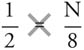(2 × N) = (1 × 8)

2N = 8

and then divide by the number with the unknown (N) to get the value of N: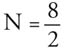N =  4

When using proportions to determine pesticide application rates, the units on the top and bottom of one ratio have to match the units on top and bottom of the other ratio.

#### Example

The label indicates that 2 lbs. of granular insecticide are to be applied per 1,000 sq. ft.

How much do you need to apply to an area that is 5,000 sq. ft.?

Express this as a proportion: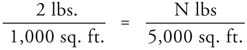Cross multiply:

1,000 x N = 2 x 5,000

1,000N = 10,000

Divide by the number with the unknown (N) to get the value of N: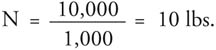Proportions can be used when converting between sq. ft. and acres.

#### Example

The label rate is 3 oz. of pesticide per 1,000 sq. ft.

How much pesticide is needed to treat 2.5 acres?

Match the units by converting your area to square feet: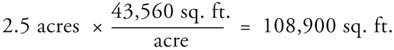## Express this as a proportion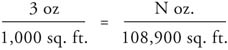Cross multiply:

1,000 x N = 3 x 108,900

1,000N = 326,700

Divide to get the value of N: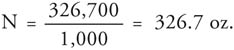Likewise, proportions can be used to convert from acres to sq. ft.

#### Example

The label rate is 3 quarts herbicide per acre, how much is needed to treat 4,200 sq. ft.?

As a first step, convert the label rate to match the square foot units

(1 acre = 43,560 sq. ft.)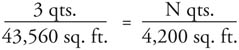Cross multiply:

43,560 x N = 3 x 4,200

43,560N = 12,600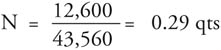As a last step, convert quarts to ounces: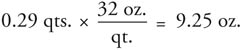## Multiplying Fractions

Many types of calculations, especially those involving conversions from one unit of measure to another, can be done by multiplying fractions or ratios. In this approach to solving problems, you need to target the results to the units of measure desired in your answer, then use ratios to cancel out the units that are the same on top and bottom.

#### Example

If 67 ounces of water were collected from a nozzle in one minute, calculate the flow rate in gallons per minute. First determine the target measurement units in your answer.  In this case, it’s gallons/minute.

## To convert from ounces to gallons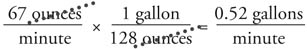Ounces are cancelled out, resulting in gallons per minute in the final answer.

#### Example

How many acres can be treated from a spray tank with a capacity of 300 gallons, if the rate of application is 20 gallons per acre?

The target units of measure in the answer are acres/tank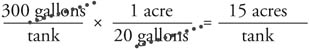Gallons are cancelled, to provide an answer in acres per tank

## Working with percentages

Many pesticides applied as spot treatments are mixed with water at a specific “percent solution.” To create this solution, convert the percentage of concentrate in the final solution to a decimal before calculating the amount of concentrate to mix with water. To convert percentages to decimals, divide the percentage number by 100 to get the decimal. This is equivalent to moving the decimal point two places to the left and adding zeroes as needed.

#### Example

Mix 3 gallons of a 2% pesticide solution in water.

1. Convert 2% to its decimal equivalent: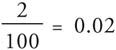2. To determine the number of ounces of pesticide needed per gallon:
Multiply the rate per gallon (0.02) by 128 (ounces in 1 gallon):0.02 × 128 = 2.6 oz.
3. To determine the amount of pesticide needed: Multiply the amount of pesticide per gallon by the total spray mixture: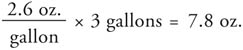4. Mix a little less than 8 ounces of pesticide with slightly less than 3 gallons of water to make a 2% solution.
Adjuvants are typically added to pesticide mixtures on the basis of a percentage of the final solution.

#### Example

How much adjuvant should be added to 400 gallons of herbicide mixture if the adjuvant is used at 0.5 percent concentration by volume?

Convert to decimal: 0.5% = 0.005

400 gal. × 0.005 = 2 gal. adjuvant

Important note on making pesticide mixtures or solutions: Pesticides and other ingredients of a liquid mixture should be considered a part of the total solution. For example, if you want to make 100 gallons of a 10% solution of a pesticide solution in water, you would add 10 gallons of pesticide concentrate to 90 gallons of water (10% of 100=10). If you added 10 gallons of pesticide to 100 gallons of water, the solution would be too dilute.

## Converting Between Active Ingredient and Commercial Product

Many recommendations state the application rate in amount of active ingredient (a.i.) per acre or 1,000 sq. ft. The active ingredient must then be converted to the actual product. For dry formulations such as wettable powders, granules and dusts, the amount of a.i. is expressed as a percentage of the weight. BrandX 75WP tells that 75% of the product weight is a.i.

A 50-pound bag of BrandX 75WP will have 37.5 pounds of active ingredient (50 × 0.75 = 37.5).

For liquid formulations such as emulsifiable concentrates and flowables, the amount of active ingredient is expressed as the weight in pounds a.i. per gallon of product. BrandZ 4EC will have 4 pounds a.i. in each gallon. This information is also provided as part of the ingredient statement, for example, “this product contains 4 pounds of active ingredient per gallon”.

A 2.5 gallon container of BrandZ 4EC has 10 lbs. of a.i. (2.5 gallons × 4 lbs. a.i./gallon = 10 lbs.).

You will need to use the right formula to calculate the amount of product needed to treat your area at the rate specified on the label. Keep in mind that the package information on dry formulations and liquid formulations means different things, and that the formula you will use to figure the amount of product will be different.

## Formula to use for dry formulations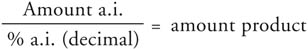#### Example

A recommendation for BrandX 75WP insecticide is to apply it at a rate of 1 lb a.i./Acre.

How much product is needed per acre?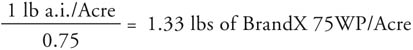How much is needed to treat 16 acres?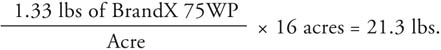## Formula to use for liquid formulations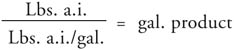#### Example

A recommendation for BrandZ 4EC fungicide is to apply it at a rate of 1 lb a.i./Acre.

How much product is needed per acre?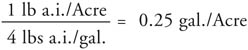How much is needed to treat 16 acres?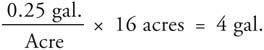Compiled by Wayne Buhler, PhD.